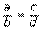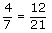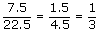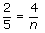Definition Of Proportion

A proportion is an equation written in the formstating that two ratios are equivalent.

In other words, two sets of numbers are proportional if one set is a constant times the other.

Example of Proportionare examples of proportions.

Solved Example on Proportion

Ques: A cardboard model of a Honda bike is part of an outdoor display. Its height is 4 ft. The actual Honda bike is 5 ft long and 2 ft high. Find the length of the model, if its dimensions are proportionate to the real bike.

Choices:

A. 11 ft
B. 12 ft
C. 9 ft
D. 10 ft
Step 2:[Write a proportion.]